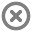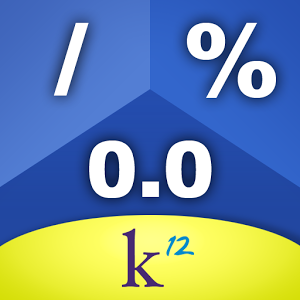Loading...## Info## Error# K12 Equivalence Tiles

by K12 Inc

Application ( 9MB )

Free

## Description

K12 Equivalence Tiles lets you compare the values of fractions, decimals, and percents up to 1 by using draggable tiles of varying values.
Fractions, decimals, and percents are used in everyday life—this app is a quick way for students to understand how they relate simply by dragging and dropping tiles of equivalent value. K12 Equivalence Tiles is not a calculator; it is a tool for students who have trouble understanding rational number equivalence (for example, 0.5, 1/2, 50% are all equal). This tool also allows students to visually perform simple operations like addition, subtraction, comparison, and ordering.

Features:
- Simple drag-and-drop controls for grade 3–12 students, parents, and teachers
- Proportionally sized tiles visually demonstrate equivalence
- Multiple rows to compare several fractions, decimals, or percents at once
- Fraction tiles of 1/1, 1/2, 1/3, 1/4, 1/6, 1/8, 1/10, and 1/12
- Decimal tiles of 1.0, 0.5, 0.33, 0.25, 0.2, 0.166, 0.125, 0.1, and 0.083
- Percent tiles of 100%, 50%, 33.3%, 25%, 20%, 16.6%, 12.5%, 10%, and 8.3%
- Equivalence chart shows how fractions, decimals, and percents relate to each other
- “Clear All” icon allows users to clear the entire workspace with a simple click
- Move draggable tiles to trash can individually to remove selected tiles from the workspace

Key Objectives and Standards:
- Big Idea: Equivalence is a fundamental property of rational numbers; equivalent fractions, percents, and decimals all name the same relationship between two values.
- Grade 3 Common Core Standards: 3.NF.1, 3.NF.2, 3.NF.3
-- Develop understanding of fractions as numbers.
- Grade 4 Common Core Standards: 4.NF.2., 4.NF.3, 4.NF.5, 4.NF.6, 4.NF.7
--Extend understanding of fraction equivalence and ordering.
--Build fractions from unit fractions by applying and extending previous understandings of operations on whole numbers.
--Understand decimal notation for fractions, and compare decimal fractions.